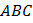# The linemeets the-axis atand the-axis at. The perpendicular bisector ofmeets the line throughparallel to-axis at. If the area of the triangleisthen the value ofis

## Question ID - 153594 :- The linemeets the-axis atand the-axis at. The perpendicular bisector ofmeets the line throughparallel to-axis at. If the area of the triangleisthen the value ofis

3537

 (7) Linemeets the axis atand. Midpoint ofisEquation of perpendicular bisector ofis(1) Now line throughand parallel toaxis isCo-ordinates ofwhere line (1) meetsisNow the area of triangleThe distance between the circumcentre and orthocentre of the triangle whose vertices are (0, 0), (6, 8) andis, then the value ofis